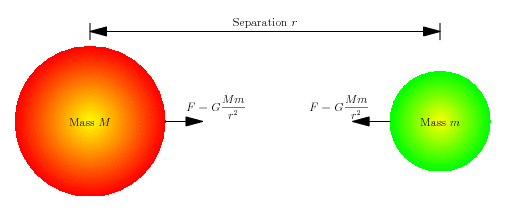•• # Law of Universal Gravitation

The law of universal gravitation gives the attraction force between two objects due to gravity.

Definition: For any pair of objects, they attract each other with a force that is directly proportional to the product of the masses of the objects and inversely proportional to the square of the distance between their centers of mass.

Gravitational constant (G): the proportionality constant is known as gravitational constant, it is a positive number and its value is about 6.67 x 10-11 N m2 kg-2.

Center of Mass: For a homogenous sphere the center of mass is just in the middle. For bodies of more complicated shapes and/or densities the center of mass is obtained integrating the product of density and position over the body (if it is possible) divided by the total mass.

Origin: The Law of Universal Gravitation was postulated by Newton in 1687 and it is part of Newtonian Mechanics. As such its applicability is limited to low speeds and bare eye distances.

Gravitation: The Law of Universal Gravitation tries to explain the phenomenon of gravitation. Gravitation is the reason why the bodies fall, why the Earth and other planets orbit around the Sun, it is the reason behind the ties and other very well documented phenomena. It can be observed right away that gravity is produced by simple aggregation of mass, the bigger the mass the stronger the field, the smaller the mass the easier to move under the effect of the bigger body. Something very particular about gravitation is that it is always attractive. The other empirical observation is that the attraction diminishes when the distance grows. The law of universal gravitation is only a formalization of the observation.

## Experimental ObservationsThe law of universal gravitation explains most of everyday phenomena such as the fall of bodies. Its main success was the prediction of the presence of Neptune based on the orbit of Uranus. Using universal gravitation, the approximated position of the planet was predicted and with that information it could be observed.

Mercury is the closest planet to the Sun and the one that suffers more its presence. The orbit shows some anomalies that can’t be explained by Newton theory. This anomaly inspired the generalization of the theory by Albert Einstein in 1915. Using its general relativity (a generalization of special relativity to address gravity) Einstein was able to explain the orbit of Mercury.

## Generalization of the Law of Universal Gravitation

The generalization of the law of universal gravitation lies under the domain of General Relativity created by the effort of Albert Einstein at the beginning of the twentieth century. The theory is a consequence of adding gravitation to Special Relativity also developed by Albert Einstein.

General Relativity uses a different approach to explain the attraction of massive bodies: gravitation is not due to a force, the presence of a mass in movement curves the space. General relativity uses a rather complicated metric and the space, following the principles of Special relativity, is not three-dimensional but tetra-dimensional including the time as forth dimension.

The Newtonian law of universal gravitation can be recovered in the limit of low speeds.

## Videos Related to Law of Universal Gravitation

1. #### myah moy

31 May, 2011 at 2:38 pm

This is very nice it tells you a lot of information about law of universal gravitation. The experiment helped me a lot so know i understand this. 😀